# B TREE Implementation

Learn: In this article, we are going to study about B-trees and the various operations performed on B tree i.e. insertion in B tree and deletion from B tree. Applications of a B tree are also prescribed in this article.
Submitted by Abhishek Kataria, on June 07, 2018

## Introduction to B TREE and its operations

A B tree is designed to store sorted data and allows search, insertion and deletion operation to be performed in logarithmic time. As In multiway search tree, there are so many nodes which have left subtree but no right subtree. Similarly, they have right subtree but no left subtree. As is known, access time in the tree is totally dependent on the level of the tree. So our aim is to minimize the access time which can be through balance tree only.

For balancing the tree each node should contain n/2 keys. So the B tree of order n can be defined as:

1. All leaf nodes should be at same level.
2. All leaf nodes can contain maximum n-1 keys.
3. The root has at least two children.
4. The maximum number of children should be n and each node can contain k keys. Where, k<=n-1.
5. Each node has at least n/2 and maximum n nonempty children.
6. Keys in the non-leaf node will divide the left and right sub-tree where the value of left subtree keys will be less and value of right subtree keys will be more than that particular key.

Let us take a B-tree of order 5,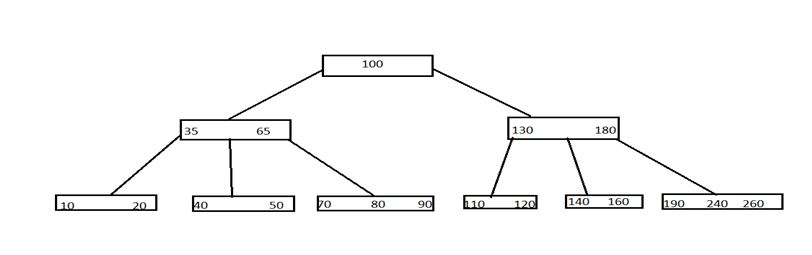Here, we can see all leaf nodes are at same level. All non-leaf nodes have no empty sub-tree and they have keys 1 less than the number of their children.

### Operations performed on B Tree

1. Insertion in B-Tree
2. Deletion from B-Tree

### 1) Insertion in B-Tree

The insertion of a key in a B tree requires the first traversal in B-tree. Through the traversal, it is easy to find that key which needs to be inserted is already existed or not. There are basically two cases for inserting the key that are:

1. Node is not full

If the leaf node in which the key is to be inserted is not full, then the insertion is done in the node.

If the node were to be full then insert the key in order into existing set of keys in the node, split the node at its median into two nodes at the same level, pushing the median element up by one level.

Let us take a list of keys and create a B-Tree: 5,9,3,7,1,2,8,6,0,4

1) Insert 52) Insert 9: B-tree insert simply calls B tree insert non-full, putting 9 to the right of 5.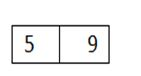3) Insert 3: Again B-tree insert non-full is called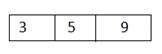4) Insert 7: Tree is full. We allocate a new empty node, make it the root, split a former root, and then pull 5 into a new root.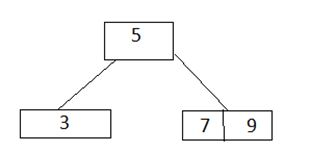5) Insert 1: It goes with 3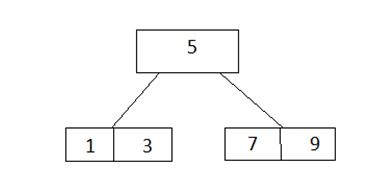6) Insert 2: It goes with 3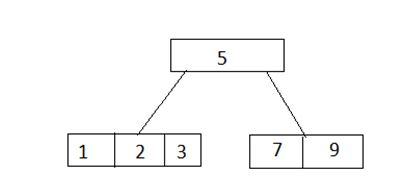7) Insert 8, 6: As firstly 8 goes with the 9 and then 6 would go with 7, 8, 9 but that node is full. So we split it bring its middle child into the root.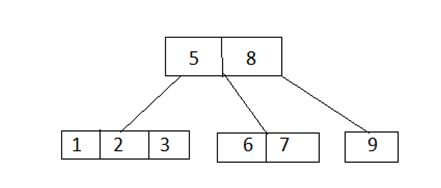8) Insert 0, 4: 0 would go with the 1, 2, and 3 which are full, so we split it sending the middle child up to the root. Now it would be nice to just stick 4 in with 3, but the B-tree algorithm requires us to split the full root. Now we can insert 4 assured that future insertion will work.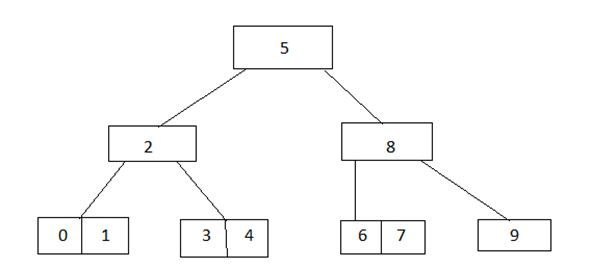### 2) Deletion from B Tree

Deletion of the key also requires the first traversal in B tree, after reaching on a particular node two cases may be occurred that are:

1. Node is leaf node
2. Node is non leaf node

Example: Let us take a B tree of order 5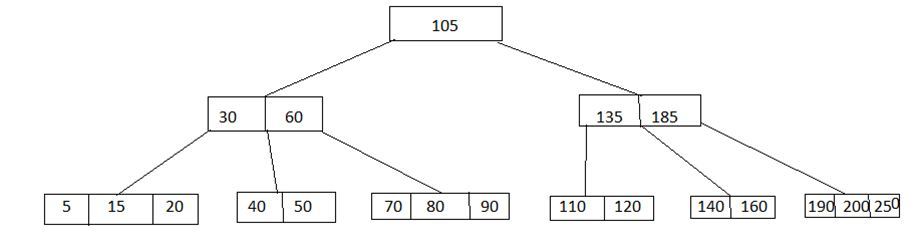1) Delete 190: Here 190 is in leaf node, so delete it from only leaf node.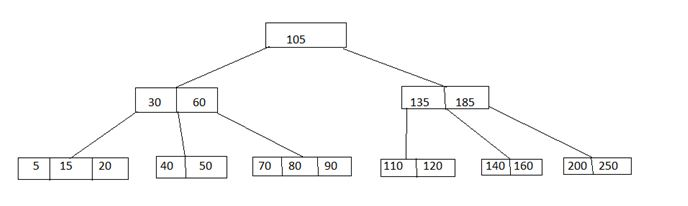2) Delete 60: Here 60 is in non leaf node. So first it will be deleted from the node and then the element of the right child will come in that node.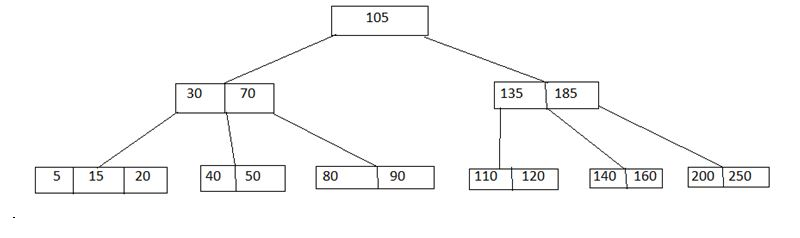### Applications of B Tree

The main application of a B tree is the organization of a huge collection of a data into a file structure. In this insertion, deletion and modification can be carried out perfectly and efficiently.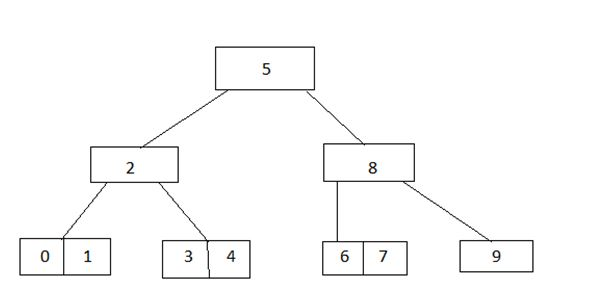Full, so we split it sending the middle child up to the root. Now it would be nice to just stick 4 in with 3, but the B-tree algorithm requires us to split the full root. Now we can insert 4 assured that future insertion will work.